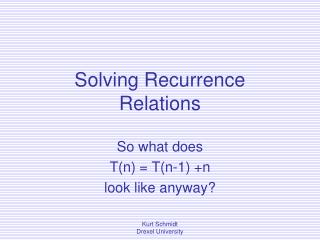DownloadDownload PresentationSolving Recurrence Relations

# Solving Recurrence Relations

Télécharger la présentation## Solving Recurrence Relations

- - - - - - - - - - - - - - - - - - - - - - - - - - - E N D - - - - - - - - - - - - - - - - - - - - - - - - - - -
##### Presentation Transcript

1. Solving RecurrenceRelations So what does T(n) = T(n-1) +n look like anyway? Kurt Schmidt Drexel University

2. Recurrence Relations • Can easily describe the runtime of recursive algorithms • Can then be expressed in a closed form (not defined in terms of itself) • Consider the linear search: Kurt Schmidt Drexel University

3. Eg. 1 - Linear Search • Recursively • Look at an element (constant work, c), then search the remaining elements… • T(n) = T( n-1 ) + c • “The cost of searching n elements is the cost of looking at 1 element, plus the cost of searching n-1 elements” Kurt Schmidt Drexel University

4. Linear Seach (cont) Caveat: • You need to convince yourself (and others) that the single step, examining an element, *is* done in constant time. • Can I get to the ith element in constant time, either directly, or from the (i-1)th element? • Look at the code Kurt Schmidt Drexel University

5. Methods of Solving Recurrence Relations • Substitution (we’ll work on this one in this lecture) • Accounting method • Draw the recursion tree, think about it • The Master Theorem* • Guess at an upper bound, prove it * See Cormen, Leiserson, & Rivest, Introduction to Algorithms Kurt Schmidt Drexel University

6. Linear Search (cont.) • We’ll “unwind” a few of these T(n) = T(n-1) + c (1) But, T(n-1)= T(n-2) + c, from above Substituting back in: T(n) = T(n-2) + c + c Gathering like terms T(n) = T(n-2) + 2c (2) Kurt Schmidt Drexel University

7. Linear Search (cont.) • Keep going: T(n) = T(n-2) + 2c T(n-2) = T(n-3) + c T(n) = T(n-3) + c + 2c T(n) = T(n-3) + 3c (3) • One more: T(n) = T(n-4) + 4c (4) Kurt Schmidt Drexel University

8. Looking for Patterns • Note, the intermediate results are enumerated • We need to pull out patterns, to write a general expression for the kth unwinding • This requires practise. It is a little bit art. The brain learns patterns, over time. Practise. • Be careful while simplifying after substitution Kurt Schmidt Drexel University

9. Eg. 1 – list of intermediates Kurt Schmidt Drexel University

10. Linear Search (cont.) • An expression for the kth unwinding: T(n) = T(n-k) + kc • We have 2 variables, k and n, but we have a relation • T(d) is constant (can be determined) for some constant d (we know the algorithm) • Choose any convenient # to stop. Kurt Schmidt Drexel University

11. Linear Search (cont.) • Let’s decide to stop at T(0). When the list to search is empty, you’re done… • 0 is convenient, in this example… Let n-k = 0 => n=k • Now, substitute n in everywhere for k: T(n) = T(n-n) + nc T(n) = T(0) + nc = nc + c0 = O(n) ( T(0) is some constant, c0 ) Kurt Schmidt Drexel University

12. Binary Search • Algorithm – “check middle, then search lower ½ or upper ½” • T(n) = T(n/2) + c where c is some constant, the cost of checking the middle… • Can we really find the middle in constant time? (Make sure.) Kurt Schmidt Drexel University

13. Binary Search (cont) Let’s do some quick substitutions: T(n) = T(n/2) + c (1) but T(n/2) = T(n/4) + c, so T(n) = T(n/4) + c + c T(n) = T(n/4) + 2c (2) T(n/4) = T(n/8) + c T(n) = T(n/8) + c + 2c T(n) = T(n/8) + 3c (3) Kurt Schmidt Drexel University

14. Binary Search (cont.) Kurt Schmidt Drexel University

15. Binary Search (cont) • We need to write an expression for the kth unwinding (in n & k) • Must find patterns, changes, as i=1, 2, …, k • This can be the hard part • Do not get discouraged! Try something else… • We’ll re-write those equations… • We will then need to relate n and k Kurt Schmidt Drexel University

16. Binary Search (cont) Kurt Schmidt Drexel University

17. Binary Search (cont) • After k unwindings: T(n) = T(n/2k) + kc • Need a convenient place to stop unwinding – need to relate k & n • Let’s pick T(0) = c0 So, n/2k = 0 => n=0 Hmm. Easy, but not real useful… Kurt Schmidt Drexel University

18. Binary Search (cont) • Okay, let’s consider T(1) = c0 • So, let: n/2k= 1 => n = 2k => k = log2n = lg n Kurt Schmidt Drexel University

19. Binary Search (cont.) • Substituting back in (getting rid of k): T(n) = T(1) + c lg(n) = c lg(n) + c0 = O( lg(n) ) Kurt Schmidt Drexel University